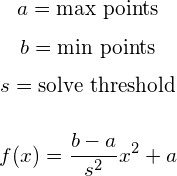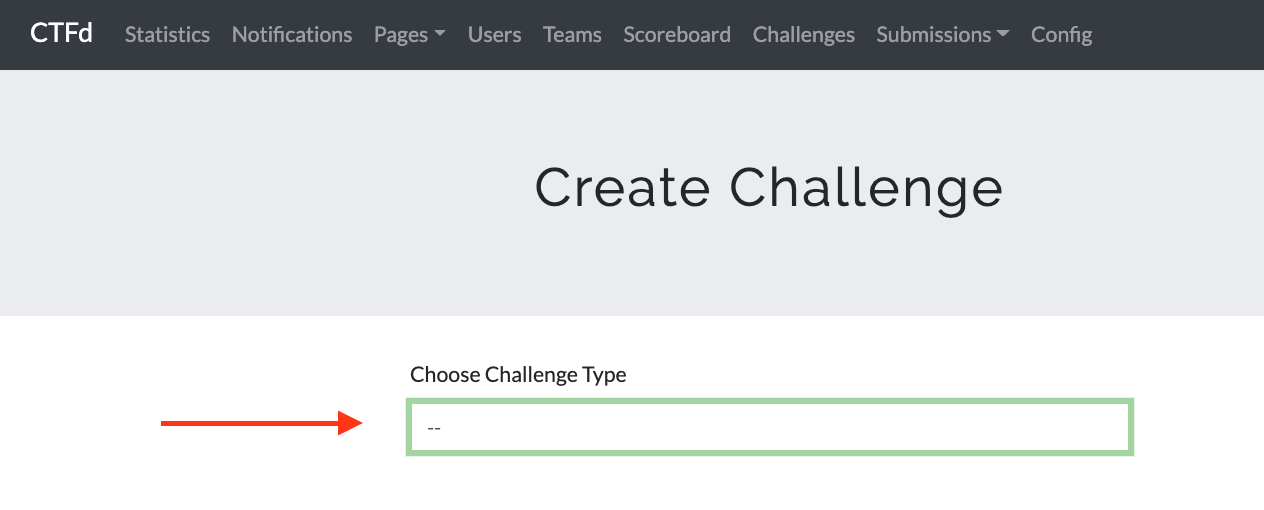# Creating Dynamic Value Challenges

## What is a Dynamic Value Challenge

A dynamic value challenge is a challenge whose point value decreases after each solve. By reducing the value of the challenge on each solve, all users who have previously solved the challenge will have lowered scores. Thus an easier and more solved challenge will naturally have a lower point value than a harder and less solved challenge.

Within CTFd you are free to mix and match regular and dynamic challenges.

The current implementation requires the challenge to keep track of three values:

• Initial - The original point valuation
• Decay - The amount of solves before the challenge will be at the minimum
• Minimum - The lowest possible point valuation

The value decay logic is implemented with the following math:or in pseudo code:

``````value = (((minimum - initial) / (decay ** 2)) * (solve_count ** 2)) + initial
value = math.ceil(value)
``````

If the number generated is lower than the minimum, the minimum is chosen instead.

CTFd uses a parabolic function instead of an exponential or logarithmic decay function so that higher valued challenges have a slower drop from their initial value.

If you would like to test the decay function, you can click here!

## How to create a Dynamic Value Challenge

In order to create a challenge you must be an admin.

1. Go to the admin panel by clicking the Admin button on the top right.

2. Click on the Challenges tab1. Select the “dynamic” challenge type from the dropdown menu2. Enter values for the challenge’s base points (from where it will start to drop off from), then add a decay rate (a larger number will drop the points faster), and finally select a minimum point value for the challenge.